# 12 7 Circles in the Coordinate Plane Warm

• Slides: 13
Download presentation12 -7 Circles in the Coordinate Plane Warm Up Use the Distance Formula to find the distance, to the nearest tenth, between each pair of points. 1. A(6, 2) and D(– 3, – 2) 9. 8 2. C(4, 5) and D(0, 2) 5 Holt Mc. Dougal Geometry12 -7 Circles in the Coordinate Plane Objectives Write equations and graph circles in the coordinate plane. Holt Mc. Dougal Geometry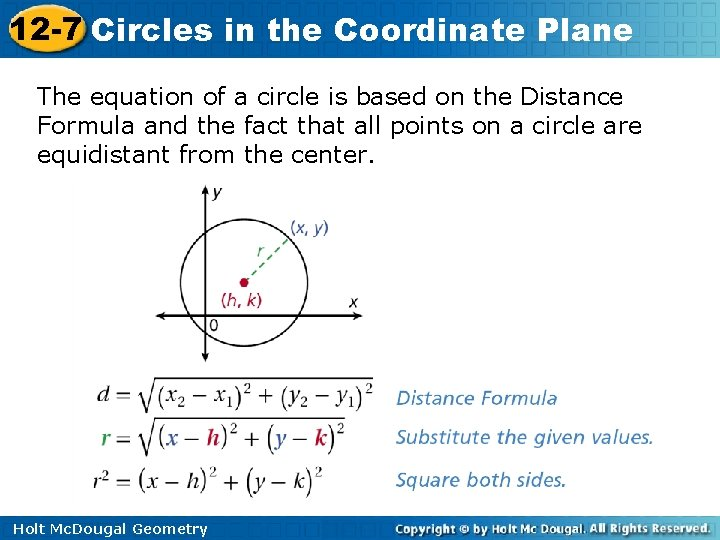12 -7 Circles in the Coordinate Plane The equation of a circle is based on the Distance Formula and the fact that all points on a circle are equidistant from the center. Holt Mc. Dougal Geometry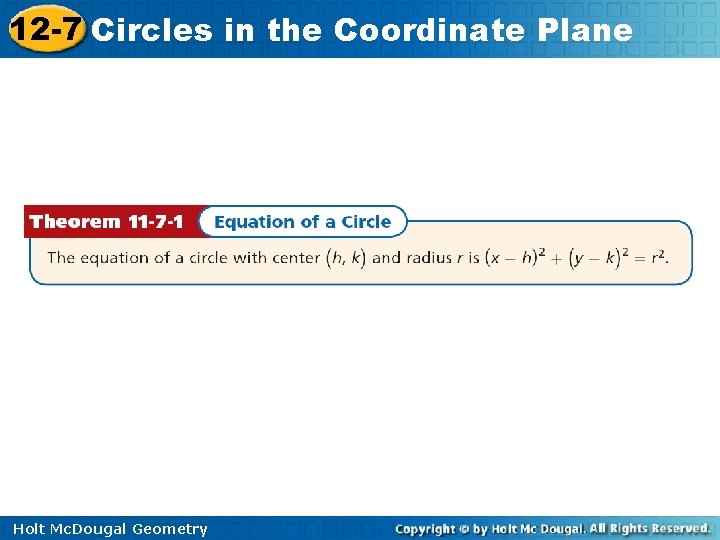12 -7 Circles in the Coordinate Plane Holt Mc. Dougal Geometry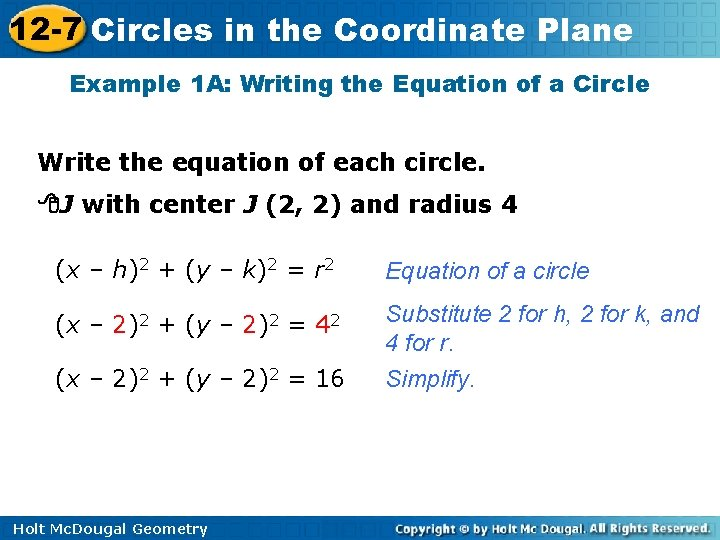12 -7 Circles in the Coordinate Plane Example 1 A: Writing the Equation of a Circle Write the equation of each circle. J with center J (2, 2) and radius 4 (x – h)2 + (y – k)2 = r 2 Equation of a circle (x – 2)2 + (y – 2)2 = 42 Substitute 2 for h, 2 for k, and 4 for r. Simplify. (x – 2)2 + (y – 2)2 = 16 Holt Mc. Dougal Geometry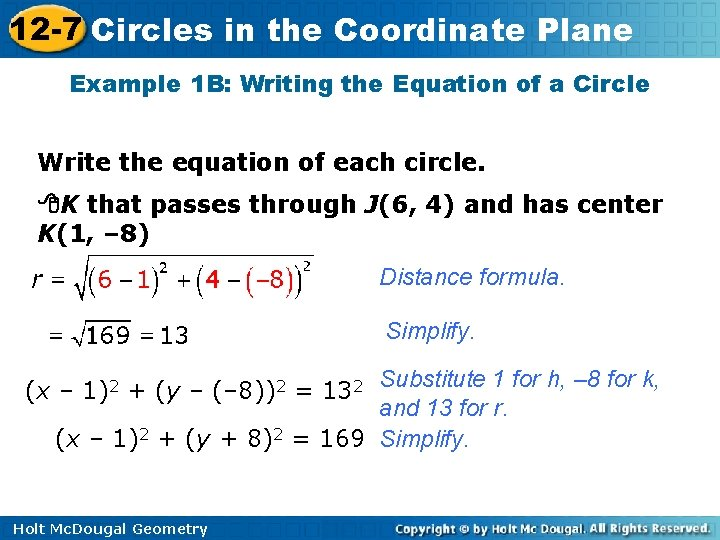12 -7 Circles in the Coordinate Plane Example 1 B: Writing the Equation of a Circle Write the equation of each circle. K that passes through J(6, 4) and has center K(1, – 8) Distance formula. Simplify. Substitute 1 for h, – 8 for k, and 13 for r. (x – 1)2 + (y + 8)2 = 169 Simplify. (x – 1)2 + (y – (– 8))2 = 132 Holt Mc. Dougal Geometry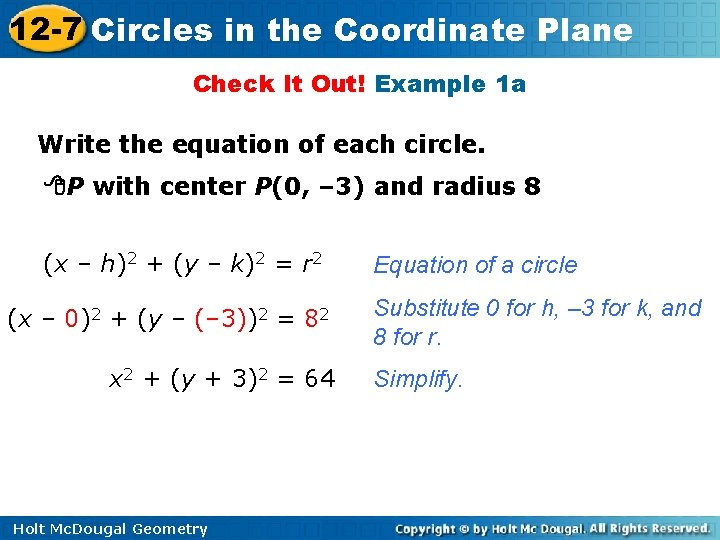12 -7 Circles in the Coordinate Plane Check It Out! Example 1 a Write the equation of each circle. P with center P(0, – 3) and radius 8 (x – h)2 + (y – k)2 = r 2 (x – 0)2 + (y – (– 3))2 = 82 x 2 + (y + 3)2 = 64 Holt Mc. Dougal Geometry Equation of a circle Substitute 0 for h, – 3 for k, and 8 for r. Simplify.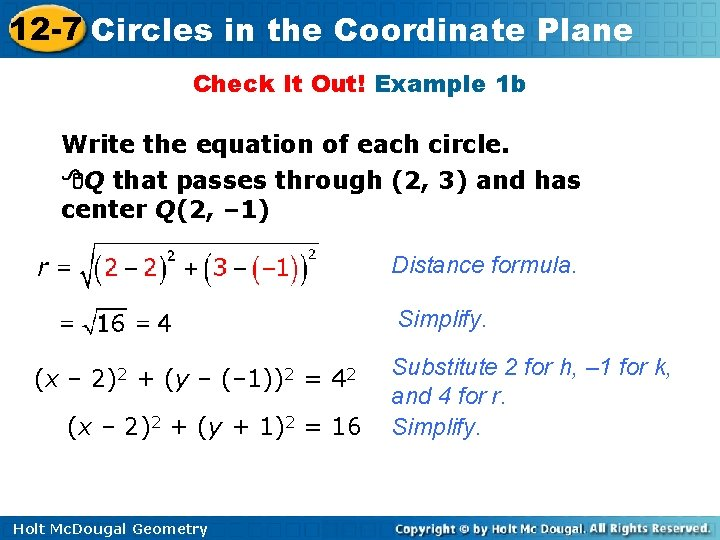12 -7 Circles in the Coordinate Plane Check It Out! Example 1 b Write the equation of each circle. Q that passes through (2, 3) and has center Q(2, – 1) Distance formula. Simplify. (x – 2)2 + (y – (– 1))2 = 42 (x – 2)2 + (y + 1)2 = 16 Holt Mc. Dougal Geometry Substitute 2 for h, – 1 for k, and 4 for r. Simplify.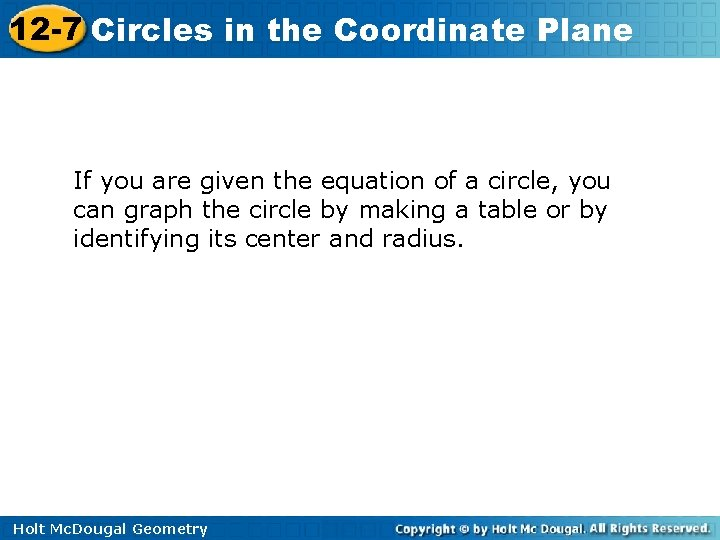12 -7 Circles in the Coordinate Plane If you are given the equation of a circle, you can graph the circle by making a table or by identifying its center and radius. Holt Mc. Dougal Geometry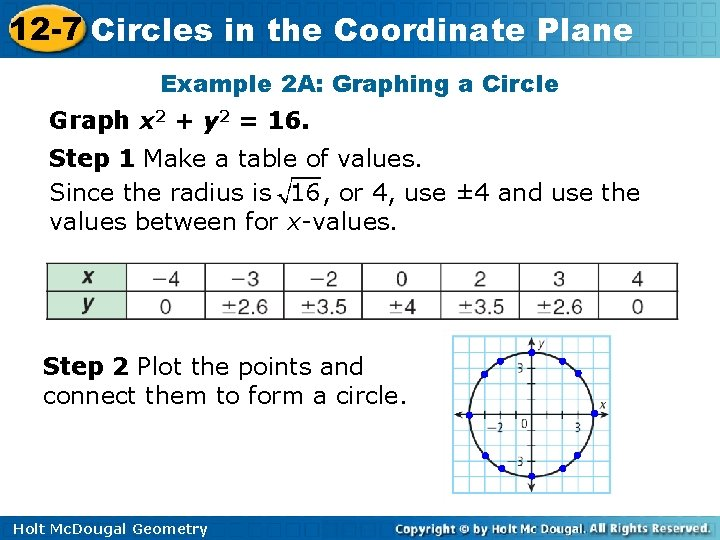12 -7 Circles in the Coordinate Plane Example 2 A: Graphing a Circle Graph x 2 + y 2 = 16. Step 1 Make a table of values. Since the radius is , or 4, use ± 4 and use the values between for x-values. Step 2 Plot the points and connect them to form a circle. Holt Mc. Dougal Geometry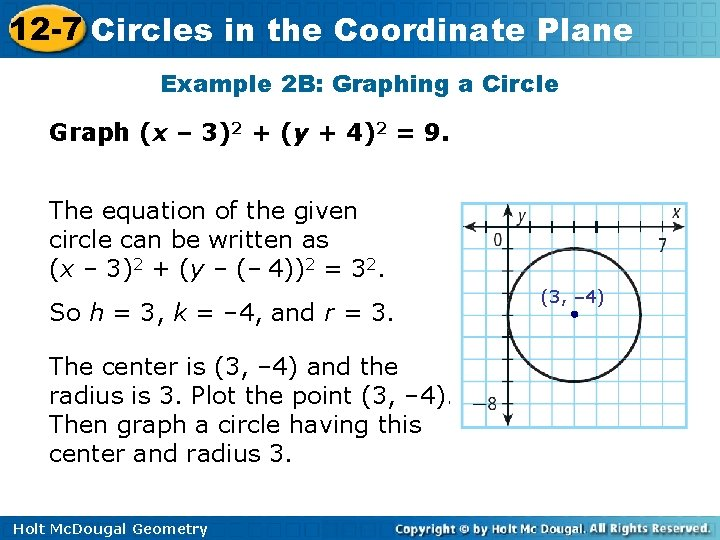12 -7 Circles in the Coordinate Plane Example 2 B: Graphing a Circle Graph (x – 3)2 + (y + 4)2 = 9. The equation of the given circle can be written as (x – 3)2 + (y – (– 4))2 = 32. So h = 3, k = – 4, and r = 3. The center is (3, – 4) and the radius is 3. Plot the point (3, – 4). Then graph a circle having this center and radius 3. Holt Mc. Dougal Geometry (3, – 4)12 -7 Circles in the Coordinate Plane Check It Out! Example 2 a Graph x² + y² = 9. Since the radius is , or 3, use ± 3 and use the values between for x-values. x 3 y 0 2 1 2. 2 2. 8 0 3 – 1 2. 8 2. 2 Step 2 Plot the points and connect them to form a circle. Holt Mc. Dougal Geometry – 2 – 3 0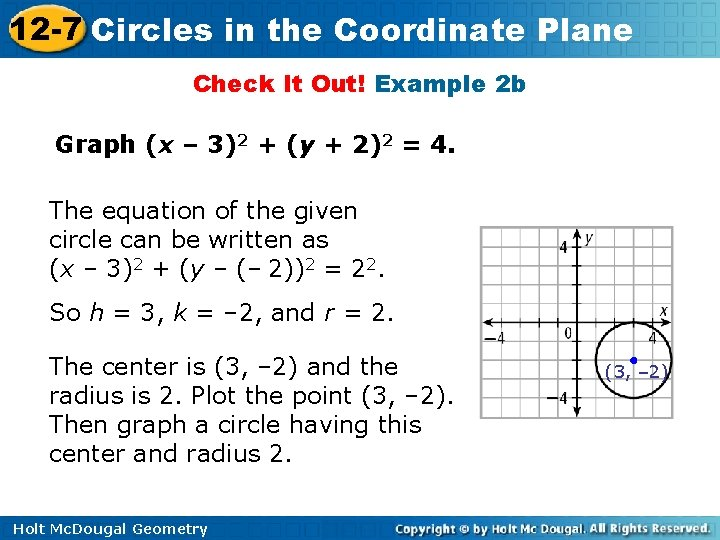12 -7 Circles in the Coordinate Plane Check It Out! Example 2 b Graph (x – 3)2 + (y + 2)2 = 4. The equation of the given circle can be written as (x – 3)2 + (y – (– 2))2 = 22. So h = 3, k = – 2, and r = 2. The center is (3, – 2) and the radius is 2. Plot the point (3, – 2). Then graph a circle having this center and radius 2. Holt Mc. Dougal Geometry (3, – 2)